# Positive and Negative Numbers

Positive numbers are defined as numbers greater than zero, or to the right of zero on the number line. Note that positive numbers include integers, but also fractional numbers and irrational numbers, such as the square root of 2, which has an infinite number of nonrepeating digits.

Negative numbers, conversely, are the numbers less than zero and on the left side of the number line.

Since the definitions are relative to zero, zero itself is neither positive nor negative. Therefore, you cannot be assured on the GMAT that a particular number is positive or negative, unless you happen to know that it’s nonzero. But you can be certain that a number is either positive, negative, or zero.

These simple rules are useful on the GMAT: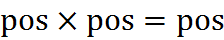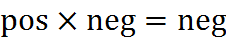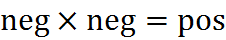Just as the even/odd multiplication rules hinged on the factor 2, these multiplication rules hinge on the factor -1. Every negative number can be thought of as having some positive factors and exactly one negative factor, -1. When a positive number and a negative number are multiplied, the product has that one -1, so it’s negative. Meanwhile, when two negative numbers are multiplied, the product has two -1’s, which multiply to 1, so the product of any two negative numbers is positive.

The division rules are similar…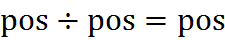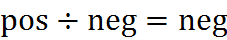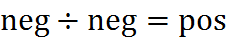… and by a similar logic. In this case, when a negative is divided by a negative, there is a -1 in the numerator and a -1 in the denominator, and they cancel.

The addition rules are not so simple: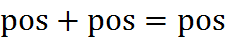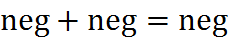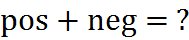There is no regular rule for the last case: whichever number has the larger absolute value will win the day.

## Practice Questions

Quotients of Positive and Negative Numbers:
http://www.gmatfree.com/quotients-of-positive-and-negative-numbers

Powers of Negative 1:
http://www.gmatfree.com/powers-of-negative-1/

Welcome! You are encouraged to register with the site and login (for free). When you register, you support the site and your question history is saved.